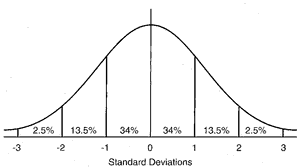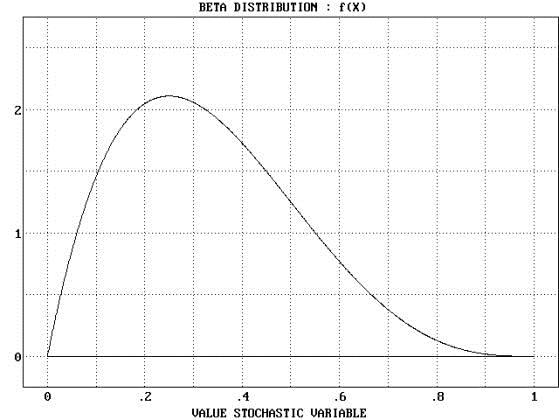# Three Point Estimates in Six Sigma and PMI

Home > Blogs > PMP Project Management > Three Point Estimates in Six Sigma and PMI

## Three Point Estimates in Six Sigma and PMI

2Both PMI and Six-Sigma Certifications rely on an understanding of statistics and in particular very basic terms, formulas and distributions. The topic generally leads to multiple examples, assistance with labs and sample questions, as well as after hours tutoring.

So I decided to write a blog to which students might refer when they need a boost or a review.  While looking at several references for CAPM, PMP, and Six Sigma Distributions, I found a prolific volume of confusing information. Confusing, at least, for anyone who does not have a background or an interest in statistics.

First, a few basics.

Testing leads to data samples. There are many goals for testing data. Two common goals for project managers and Six-sigma practitioners are: finding the root cause of an issue by mapping the set of symptoms, or achieving execution conformance so that a desired outcome might be consistently achieved. Test samples might be random, scattered but correlated, grouped or clustered, linear or curvilinear, or related through more complex associations.

The PMBOK™ 5th Edition references multiple Quality tools that may be used to analyze data including the cause-and-effect (Ishikawa) diagram, check sheet, control chart, histogram, Pareto chart, flow chart, and scatter diagram.

When the data sample demonstrates a linear or curvilinear distribution, we can apply more advanced statistics to acquire both deterministic (cause) and predictive (forecasting) results. A test result population plotted on a histogram (bar chart) can also result in a curve drawn through or over the histogram data set(s). The curve’s shape, center, and spread can be used to apply mathematical analysis.

For the CAPM, PMP, and Green-belt Lean Six Sigma certification, the emphasis on shape and the relevant formulas and relationships are limited to the normal distribution or bell curve.

NOTE: I discovered multiple references to the beta distribution while researching this article. Similar statistical analysis may be applied to a beta distribution, but it does not bear the same characteristics as a normal distribution such that some of the assumptions used to solve normal distributions do NOT apply. For instance, the three-point estimating technique used for PERT does NOT consistently apply to a beta distribution.

Some more terms.

Mean. (Not the emotion or human characteristic). The mathematical average of a population. Calculated by totaling the values for all of the data samples and dividing by the number of samples. Often called the average.

Median. The mid-point of a population or sample curve. Count the number of samples, then start at either end of the curve and count to the middle.  Remember all those ‘curve breakers’ in school? The one’s with results way above or below the average? They shifted the curve so that the median and the mean are not the same value.

Mode. The data point that occurs most often in the sample set. In the data string : 1, 2,2, 3,3,4,4,4,4,5,5,6  the number 4 would be the mode because it occurs the most frequently.

Variance. The difference between two values. In statistics the Variance is a little more complicated. It is calculated as the average of the squared differences from the mean. (So take the difference between the mean and each sample, then square the results, then work out the average of all the squared values … sum them all then divide by the number of samples. Go find a computer and use a spreadsheet or a program for this one…)

Standard Deviation.  The square root of the statistical variance. The symbol is the lower case Greek letter Sigma – σ . The standard deviation tells us about the spread of the curve. In a normal distribution, the standard deviation follows a recognizable pattern and allows for both simple and clear calculations.

Normal distribution

The normal distribution is often referred to as a ‘bell curve’. The population samples are evenly distributed on either side of the mid point, such that the mean (average) is statistically identical to the median (middle number) and the mode (most common number) in the population. The standard deviation follows a pattern that enables the statistician to easily determine population patterns with limited information.The normal distribution is typically displayed with six standard deviations as shown in the drawing above.

In the CAPM/PMPC course [http://www.interfacett.com/training/pmpc-project-management-fundamentals-and-professional-certification/], I suggest that all test candidates memorize the following distribution populations as provided in the PMBOK™ 5th Edition:

• + 1 σ = 68.26% (34% on either side of the mean)
• + 2 σ = 95.46% (34%+13.5% = 47.5% on either side of the mean)
• + 3 σ = 99.73% (34%+13.5% + 2.5% = 49% on either side of the mean)

Since the beta distribution is so often mis-characterized in test preparation materials, I provide just a little information so that you can see that is not the same shape and therefore not identical mathematics.

A beta distribution as discussed originally by Karl Pearson is a solution of a differential equation wherein the mean, median, and mode are not the same value. In other words, the values associated with the population are shifted so that the curve is more like a ‘cocked hat’, J-curve, U-curve, or other shifted and re-scaled shapes.If you are curious about other statistical distributions, J. DeLayne Stroud offers a great article in the iSixSigma site called Understanding Statistical Distributions for Six Sigma. Note that even Mr. DeLayne’s article stresses the emphasis on the normal curve as the basis for Six-Sigma green belt certification.

Okay. Back to our normal distribution.

Using the three point estimating technique, our well trained project manager interviews the work-package owner or subject matter expert to obtain time or cost estimates. In return, the respondent provides their most optimistic (O), most pessimistic (P), and Most Likely (ML) estimates for work results.P exists somewhere within the 2.5% at one end of the distribution, while O exists within the 2.5% at the other end of the distribution. ML resides somewhere within the +2 σ or -2 σ (a total of 4 σ) in the middle of the distribution.

Hence to calculate the best estimate using a three-point process, we use the following formula:

(O + 4*ML + P)/6

The result is more accurate, given that the answers really do exist within a normalized population, than a pure average of the three answers.

At this point, I feel compelled to clarify a standard misperception stated by many project managers. Even those with a PMP.

PERT, which stands for Program Evaluation and Review Technique, is a schedule planning process developed originally by the U.S. Navy in the 1950’s.  PERT uses a Precedence Diagram Method (PDM) to apply the Critical Path Methodology (CPM). PERT schedule diagrams rely on a time scale that is represented in seconds or minutes. The accuracy required for such a tight time scale requires the three-point estimating process (at a minimum – or optimally computer simulations that better tighten the standard deviation.)

You should associate three-point estimating with PERT. But three-point estimating is NOT PERT and PERT is not an accurate label for three-point estimating. A PERT chart is a schedule network diagram, not a statistical distribution or a detailed scope statement as often mis-represented.

One other formula that may be applied to the normal distribution is the ability to determine the standard deviation (σ) given only the Pessimistic (P) and Optimistic (O) estimates. Because of the even population distribution:

σ = (P-O)/6

Some Examples

We provide Aileen Ellis’ PMP Exam Simplified book with our CAPM/PMP course delivery. Aileen’s examples are as challenging as you might see on a certification exam, so I am borrowing two to demonstrate the application of the normal distribution and the three-point estimate.

You are a project manager for a logging company. This month you are charted to deliver 10,000 units that are 60 centimeters each. Your upper control limit on your process is 63 centimeters. Your lower control limit on your process is 57 centimeters. Approximately what percentage of your units will be above 61 centimeters?

Let’s solve this. We have the upper and lower control limits, which represent the two ends of a normal distribution. So P=63 and O=57. So σ (one standard deviation) = (63-57)/6 which is 6/6 or 1. σ is one centimeter. The mean is your target, which is 60 centimeters. So, using the distributions, 68% of the population will be between 59 centimeters and 61 centimeters – this is + 1 σ. How much will be above 61 cm? Everything in the +2 σ and +3 σ sections of the population. So 13.5% + 2.5%, = 16%. Using the more precise ranges provided in the PMBOK™ 5th edition the result would be 15.9%, but you would likely spot the slight difference on a test question.

Second example, also borrowed from Aileen.

The pessimistic time to complete Activity A is 22 days. The optimistic time is 10 days. The standard deviation for activity A is?

Wait a minute …. Before you read my answer, try this one yourself!

Go ahead. Grab a piece of paper and give it a try.

Really.

Okay … here is how you solve it.

One standard deviation (σ) is (P-O)/6, so (22-10)/6. This is 12/6=2. Since the units in this question are days. The standard deviation is 2 days.

I knew you could do it! And I look forward to seeing you in the classroom, or online!

Steven Fullmer
Interface Technical Training Staff Instructor

Steve teaches PMP: Project Management Fundamentals and Professional Certification, Windows 7, Windows 8.1 and CompTIA classes in Phoenix, Arizona.

## Videos You May Like

### Agile Methodology in Project Management

In this video, you will gain an understanding of Agile and Scrum Master Certification terminologies and concepts to help you make better decisions in your Project Management capabilities. Whether you’re a developer looking to obtain an Agile or Scrum Master Certification, or you’re a Project Manager/Product Owner who is attempting to get your product or … Continue reading Agile Methodology in Project Management

### JavaScript for C# Developers – September 24, 2014

Is JavaScript worth taking the time to learn if I’m a server-side .NET developer? How much of C# carries over to JavaScript? In this recorded video from Dan Wahlin’s webinar on September 24,2014, Dan answers these questions and more while also discussing similarities between the languages, key differences, and the future of JavaScript (ES6). If … Continue reading JavaScript for C# Developers – September 24, 2014

### Detailed Forensic Investigation of Malware Infections – April 21, 2015

How does an investigator hunt down and identify unknown malware? In this recording of our IT Security training webinar on April 21, 2015, Security expert Mike Danseglio (CISSP / CEH) performed several malware investigations on infected computers and identify symptoms, find root cause, and follow the leads to determine what’s happening. He demonstrated his preferred … Continue reading Detailed Forensic Investigation of Malware Infections – April 21, 2015

## Write a Comment

### See what people are saying...

1.Interface

The answer provided in the example is correct. +2 σ and +3 σ asks what would be ‘above’ or greater than the 61cm size. Both sides of the mean would identify values that do not match 61 cm, both above and below 61cm. Only the +2 σ and +3 σ sections under the curve are ABOVE 61 cm.

Steven Fullmer
Interface Instructor

2.Jayant

Hi,

I would like to highlight something regarding the below :
“How much will be above 61 cm? Everything in the +2 σ and +3 σ sections of the population. So 13.5% + 2.5%, = 16%.”

Please correct me if i am wrong, the correct answer should be 32% (16%x2..on both sides of mean). 16% on one side of the mean does make sense but it should not be correct answer for the question being asked “How much will be above 61cm”.